# What is Kleene’s Theorem in TOC?

Kleene's Theorem states the equivalence of the following three statements −

• A language accepted by Finite Automata can also be accepted by a Transition graph.

• A language accepted by a Transition graph can also be accepted by Regular Expression.

• A language accepted by Regular Expression can also be accepted by finite Automata.

## Kleene’s theorem proof part-1

A language accepted by Finite Automata can also be accepted by a Transition graph.

Consider an example Let L=aba over an alphabet {a,b}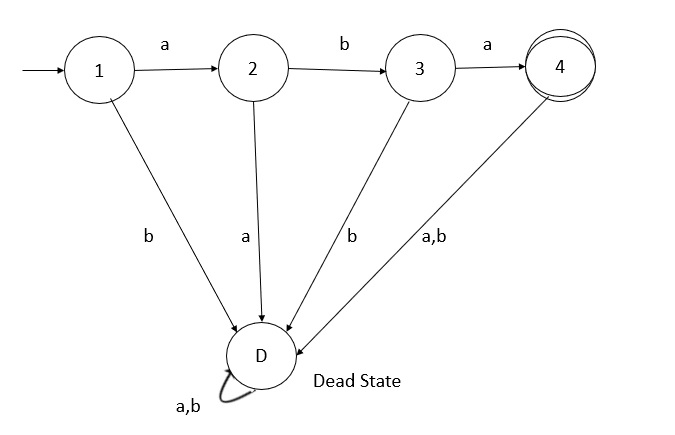## Third part of Kleene’s theorem

A language accepted by Regular Expression can also be accepted by finite Automata.

## Theorem

Any language that can be defined with RE can be accepted by some Finite State Machine (FSM) is also regular.

## Proof

The proof is by construction.

For a given RE α, we can construct a FSM M such that

L (α) = L (M)

• If α is any c € Σ, we can construct a simple FSM as follows −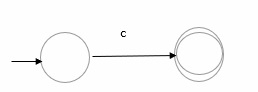• If α is φ, we construct simple FSM as follows −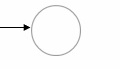• If α is €, we construct simple FSM as −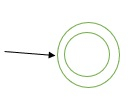Let us construct FSMs to accept language that are defined by regular expression that exploits the operations of concatenation, union and Kleene star.

Step 1

Let β and γ be RE that defined languages over the alphabet Σ

If L(β) is regular, then it is accepted by some FSM

M1 = (Q1, Σ, δ1, q1, F1)

Let L(γ) is regular, then it is accepted by some FSM

M2= (Q2, Σ, δ2, q2, F2)

Step 2

If RE α= β ∪ γ and if both L(β) and L(γ) are regular,

Then we construct M3=( Q3, Σ, δ3, q3, F3), such that

L(M3)=L(α)=L(β) ∪ L(γ)

Step 3

Let P accept L = {a} and Q accepts L = {b}, then R can be represented as a combination of P and Q by using the provided operations as −

R = P+ Q

The transition diagram for the same is given below −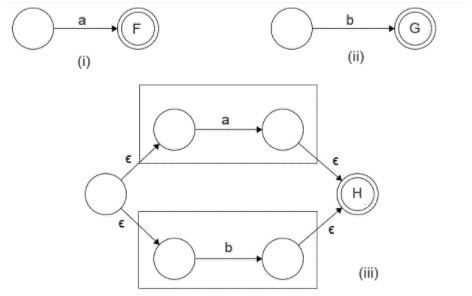We observe the following in the transition diagram −

• In case of union operation we can have a new start state. From there, a null transition proceeds to the starting state of both the Finite State Machines.

• The final states of both the Finite Automata are converted to intermediate states. The final state is unified into one which can be traversed by null transitions.